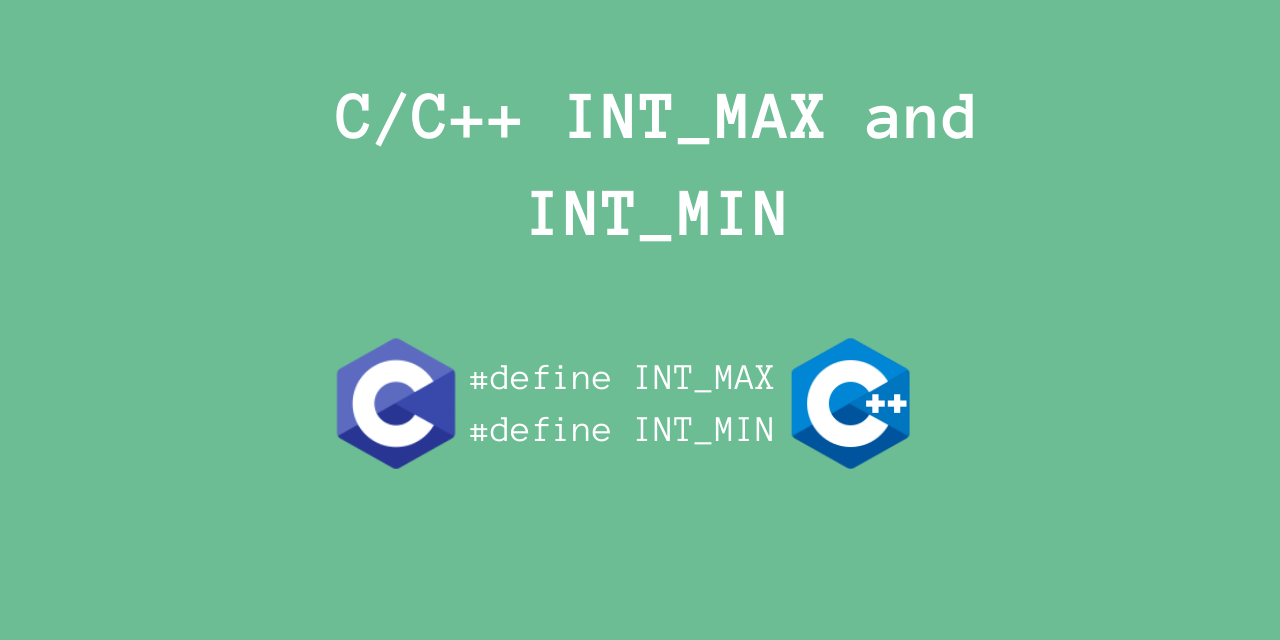# Using INT_MAX and INT_MIN in C/C++

Published on August 3, 2022By Vijaykrishna RamWhile we believe that this content benefits our community, we have not yet thoroughly reviewed it. If you have any suggestions for improvements, please let us know by clicking the “report an issue“ button at the bottom of the tutorial.

In this article, we’ll take a look at using INT_MAX and INT_MIN in C/C++.

These are actually useful macros which represent the maximum and minimum integer values.

Let’s take a look at it, using some examples.

## Using INT_MAX and INT_MIN

INT_MAX is a macro which represents the maximum integer value. Similarly, INT_MIN represents the minimum integer value.

These macros are defined in the header file `<limits.h>`, so you must include it.

``````#include <limits.h>

INT_MAX

INT_MIN
``````

Note that any integer variable must lie between INT_MIN and INT_MAX.

Typically, integers are stored as 4 bytes (32 bits).

This means that in almost all machines, the maximum integer value will be 2^(31) - 1 = +2147483647.

The minimum integer value will be -(2^31) = -2147483648

Let’s verify this, for our machine.

``````#include <stdio.h>
#include <limits.h>

int main() {
printf("Maximum Integer Value: %d\n", INT_MAX);
printf("Minimum Integer Value: %d\n", INT_MIN);
return 0;
}
``````

Output

``````Maximum Integer Value: 2147483647
Minimum Integer Value: -2147483648
``````

Indeed, we get what we predict.

Let’s now take another example, to correctly predict any integer overflow or underflow.

``````#include <stdio.h>
#include <limits.h>

int main() {
int value = 0;
while (value >= 0) {
// Check for overflow
if (value == INT_MAX) {
printf("value = %d. Possible overflow!\n", value);
}
value ++;
}
printf("Now, value = %d\n", value);
value = 0;
while (value <= 0) {
// Check for underflow
if (value == INT_MIN) {
printf("value = %d. Possible underflow!\n", value);
}
value --;
}
printf("Now, value = %d\n", value);
return 0;
}
``````

Output

``````value = 2147483647. Possible overflow!
Now, value = -2147483648
value = -2147483648. Possible underflow!
Now, value = 2147483647
``````

While this takes a good few seconds to run, this does indeed do what we expect.

The integer will overflow to `INT_MIN`, and will underflow to `INT_MAX`.

This is useful to detect such jumps in the values.

## Why do we need these macros?

Often, for certain algorithms, it is sometimes necessary to initialize a variable as the lowest/highest value.

The number of bits of the datatype may differ based on the machine.

To make the usage of the maximum/minimum values be consistent, it would be convenient if everyone could use the same macros!

This is exactly why these kinds of macros exist -

• To spare you from remember the actual values
• Have consistent programming patterns across all machines
• Very convenient to use

Hopefully, these reasons may convince you to use such kinds of macros whenever you build your own C/C++ library.